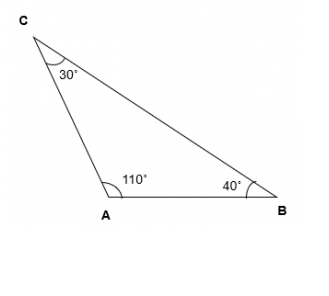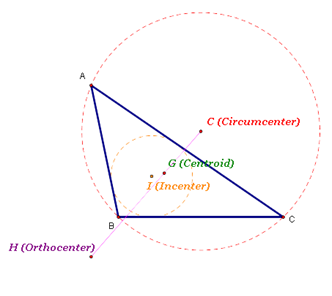• Call Now

1800-102-2727•

# Obtuse-Angled Triangle

## What is an Obtuse- Angled Triangle?

Triangles are closed 2D shapes with three sides of the same length or different lengths and three angles with the same or different measurements in geometry. Triangles are classified into six different types based on their characteristic lengths and angles. These include scalene triangles, right-angled triangles, acute-angled triangles, obtuse-angled triangles, isosceles triangles, and equilateral triangles.

An obtuse-angled triangle is a triangle in which one of the internal angles is greater than 90°. If one angle of an obtuse triangle measures over 90°, the total of the other two angles is less than 90°.According to the angle sum property:

The sum of all angles of a triangle is 180° (A+ B+ C). Since A = 110°, then B + C = 70°.

In the given figure as angle A is over 90°, the triangle ABC is an obtuse triangle. Also, the total of angle B and angle C is less than 90°. Thus it can be concluded that, if one of the triangle's angles is obtuse, then the other two angles will always be acute.

## Properties of an Obtuse- Angled Triangle

• A triangle cannot be both right-angled and obtuse-angled. Because there is only one right angle in a right-angled triangle, the other two angles are acute. As a result, an obtuse-angled triangle cannot have a right angle and vice versa.
• An obtuse triangle can either be a scalene triangle or an isosceles triangle, but it cannot be an equilateral triangle. An equilateral triangle has equal sides and each angle measures 60°.
• The longest side of the triangle is the one opposite the obtuse angle.
• The centroid and incenter are within the obtuse-angled triangle, whereas the circumcenter and orthocenter are located outside of the triangle.

### How to Recognize an Obtuse Angled Triangle?

In order to recognize if the given triangle is an obtuse-angled triangle based on the sides of the triangle, a simple formula can be used:

a2 + b2 < c2

Where, a and b are small sides of the triangle

c is the largest side opposite to the obtuse angle.

If the sum of the squares of the smaller sides of a triangle is less than the square of the largest side, then the triangle is obtuse-angled.

## Area of an Obtuse Angled TriangleAn obtuse-angled triangle has one angle greater than 90°, so we create a perpendicular line outside of the triangle where we elongate the height to get the area of an obtuse triangle. Once we have the height, we can use the formula given below to calculate the area of an obtuse triangle.

Area of ABC = ½*h*b, where BC (b) is the base and h is the triangle's height.

(A triangle has three altitudes, three vertices, and three sides. In the case of an obtuse-angled triangle, the height or altitude outside the triangle comes from the acute angles. The base can be extended to determine the height of an obtuse triangle.)

## The Perimeter of an Obtuse Angled Triangle

The perimeter of an obtuse triangle is the sum of its sides' measurements. As a result, the formula for the perimeter of an obtuse-angled triangle is:

Obtuse angled triangle perimeter = (a + b + c) units.

## Area of an Obtuse Angled Triangle by Using Heron's Formula

We can calculate the area of an obtuse-angled triangle using Heron's formula. Consider the ΔABC, with sides a, b, and c of known length.Talk to our expert
Resend OTP Timer =
By submitting up, I agree to receive all the Whatsapp communication on my registered number and Aakash terms and conditions and privacy policy